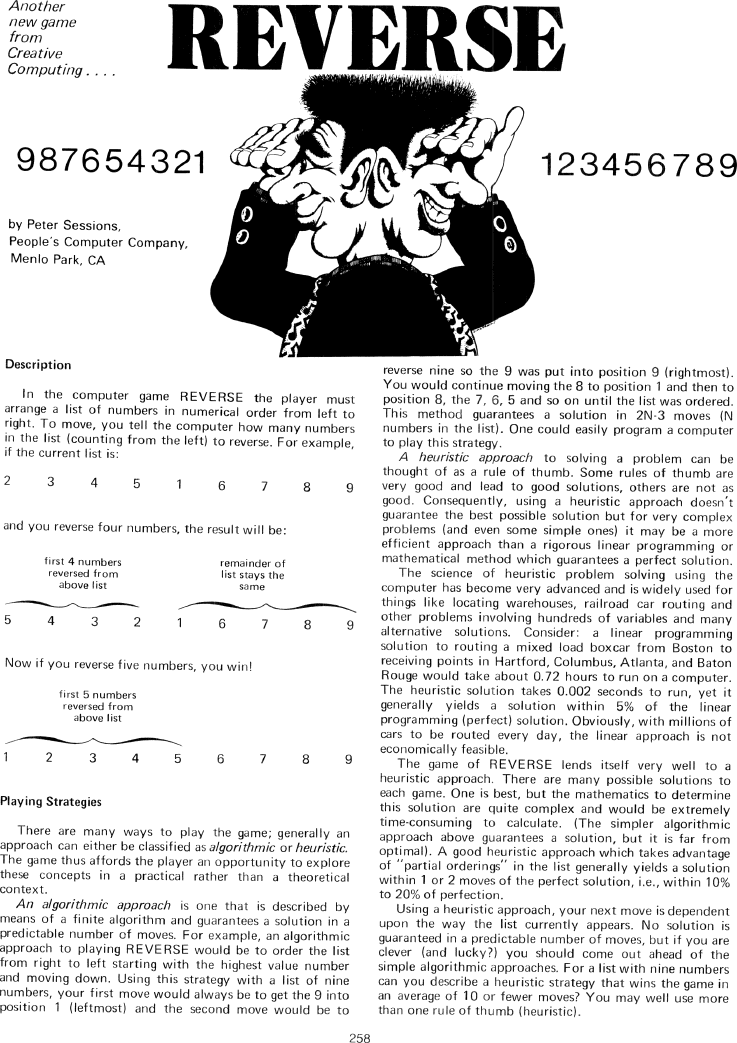The Best of Creative Computing Volume 1 (published 1976)

 `Page 258 << PREVIOUS >> NEXT Jump to page: Go to contents Go to thumbnails`

Reverse (BASIC computer game, arrange numbers, algorithmic vs. heuristic approaches to problem solving, BASIC program listing, sample run)```Another new game from Creative Computing....

REVERSE

987654321  123456789

by Peter Sessions,
People's Computer Company,
Menlo Park, CA

Description

In the computer game REVERSE the player must arrange a list of numbers in
numerical order from left to right. To move, you tell the computer how many
numbers in the list (counting from the left) to reverse. For example, if the
current list is:

2 3 4 5 1 6 7 8 9

and you reverse four numbers, the result will be:

first 4 numbers
reversed from
above list
[image] 5 4 3 2

remainder of list stays the same
[image] 1 6 7 8 9

Now if you reverse five numbers, you win!

first 5 numbers
reversed from
above list
[image] 1 2 3 4 5 6 7 8 9

***

Playing Strategies

There are many ways to play the game; generally an approach can either be
classified as algorithmic or heuristic. The game thus affords the player an
opportunity to explore these concepts in a practical rather than a theoretical
context.

An algorithmic approach is one that is described by means of a finite algorithm
and guarantees a solution in a predictable number of moves. For example, an
algorithmic approach to playing REVERSE would be to order the list from right to
left starting with the highest value number and moving down. Using this strategy
with a list of nine numbers, your first move would always be to get the 9 into
position 1 (leftmost) and the second move would be to reverse nine so the 9 was
put into position 9 (rightmost). You would continue moving the 8 to position 1
and then to position 8, the 7, 6, 5 and so on until the list was ordered. This
method guarantees a solution in 2N-3 moves (N numbers in the list). One could
easily program a computer to play this strategy.

A heuristic approach to solving a problem can be thought of as a rule of thumb.
Some rules of thumb are very good and lead to good solutions, others are not as
good. Consequently, using a heuristic approach doesn't guarantee the best
possible solution but for very complex problems (and even some simple ones) it
may be a more efficient approach than a rigorous linear programming or
mathematical method which guarantees a perfect solution.

The science of heuristic problem solving using the computer has become very
advanced and is widely used for things like locating warehouses, railroad car
routing and other problems involving hundreds of variables and many alternative
solutions. Consider: a linear programming solution to routing a mixed load
boxcar from Boston to receiving points in Hartford, Columbus, Atlanta, and Baton
Rouge would take about 0.72 hours to run on a computer. The heuristic solution
takes 0.002 seconds to run, yet it generally yields a solution within 5% of the
linear programming (perfect) solution. Obviously, with millions of cars to be
routed every day, the linear approach is not economically feasible.

The game of REVERSE lends itself very well to a heuristic approach. There are
many possible solutions to each game. One is best, but the mathematics to
determine this solution are quite complex and would be extremely time-consuming
to calculate. (The simpler algorithmic approach above guarantees a solution, but
it is far from optimal). A good heuristic approach which takes advantage of
"partial orderings" in the list generally yields a solution within 1 or 2 moves
of the perfect solution, i.e., within 10% to 20% of perfection.

Using a heuristic approach, your next move is dependent upon the way the list
currently appears. No solution is guaranteed in a predictable number of moves,
but if you are clever (and lucky?) you should come out ahead of the simple
algorithmic approaches. For a list with nine numbers can you describe a
heuristic strategy that wins the game in an average of 10 or fewer moves? You
may well use more than one rule of thumb (heuristic).

258Page 258        << PREVIOUS        >> NEXT        Jump to page:                Go to contents        Go to thumbnails

<!--